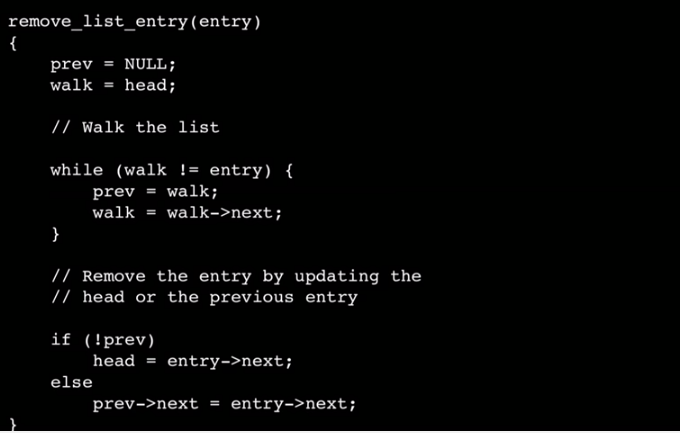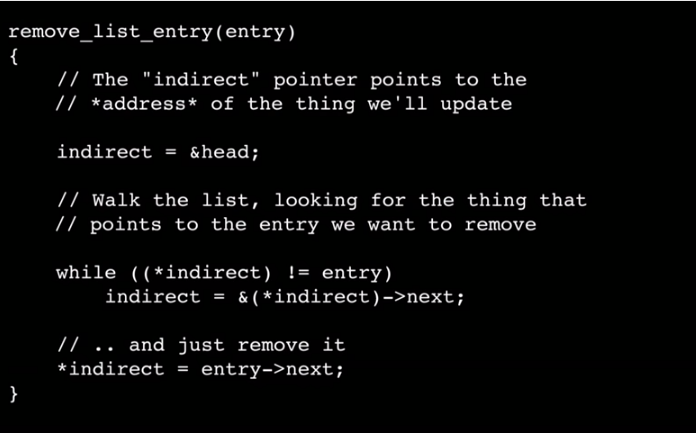# 向 Linus Torvalds 學習讓編出的代碼具有 “good taste”Linus 解釋了一下新的代碼，它消除了邊緣情況，就是這樣。然後採訪轉入了下一個話題。

```for (r = 0; r < GRID_SIZE; ++r) {
for (c = 0; c < GRID_SIZE; ++c) {

// Top Edge
if (r == 0)
grid[r][c] = 0;

// Left Edge
if (c == 0)
grid[r][c] = 0;

// Right Edge
if (c == GRID_SIZE - 1)
grid[r][c] = 0;

// Bottom Edge
if (r == GRID_SIZE - 1)
grid[r][c] = 0;
}
}
```

1. 複雜性 — 在雙層循環裡面使用 4 個條件語句似乎過於複雜。
2. 高效性 — 假設 GRID_SIZE 的值為 64，那麼這個循環需要執行 4096 次，但需要進行賦值的只有位於邊緣的 256 個點。

```for (i = 0; i < GRID_SIZE * 4; ++i) {

// Top Edge
if (i < GRID_SIZE)
grid[i] = 0;

// Right Edge
else if (i < GRID_SIZE * 2)
grid[i - GRID_SIZE][GRID_SIZE - 1] = 0;

// Left Edge
else if (i < GRID_SIZE * 3)
grid[i - (GRID_SIZE * 2)] = 0;

// Bottom Edge
else
grid[GRID_SIZE - 1][i - (GRID_SIZE * 3)] = 0;
}
```

```for (i = 0; i < GRID_SIZE; ++i) {

// Top Edge
grid[i] = 0;

// Bottom Edge
grid[GRID_SIZE - 1][i] = 0;

// Left Edge
grid[i] = 0;

// Right Edge
grid[i][GRID_SIZE - 1] = 0;
}
```